Catch up on the latest AI articlesA Quadruped Robot Controller That Can Successfully Walk In Diverse Environments!

3 main points
✔️ Achieve stable walking in various environments such as snowfield and forest
✔️ Two-stage strategy learning with the teacher and student strategies
✔️ Curriculum learning by automatically adjusting the difficulty of the terrain

written by Joonho LeeJemin HwangboLorenz WellhausenVladlen KoltunMarco Hutter
(Submitted on 21 Oct 2020)

Subjects: Robotics (cs.RO); Machine Learning (cs.LG); Systems and Control (eess.SY)code：The images used in this article are from the paper, the introductory slides, or were created based on them.

first of all

The acquisition of walking motions in various environments including unknown environments for a quadruped robot will lead to the expansion of the robot's applicable area. However, the walking control controllers that have been proposed so far have problems such as requiring human tuning and being vulnerable to unknown environments, and they are not at a level where they can be applied in practice. In addition, even the RL-based controllers were limited to relatively simple environments such as a laboratory or flat terrain.

The paper presented here proposes a controller that achieves stable walking in diverse environments such as snow, forests, stairs, and streams without individual tuning.

The proposed controller is found to be robust enough to walk on completely untrained terrain that is brittle or slippery and shows very good stability compared to the previous methods. Now, as in the original paper, I would like to look at the performance and methods in order.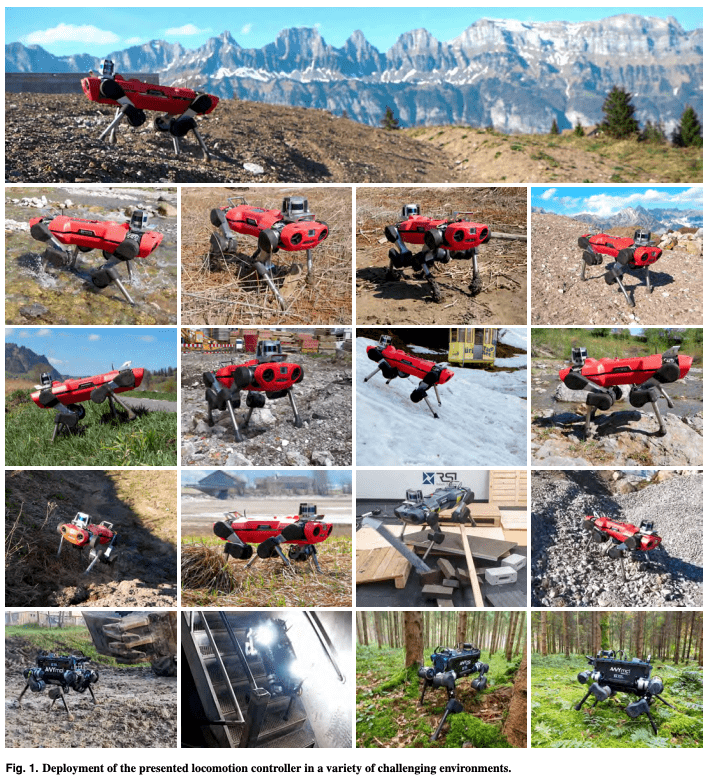performance

We will look at several different environments. Note that the conventional method (Baseline) used for comparison is the most advanced one at the time, depending on the environment.

natural (physical) environment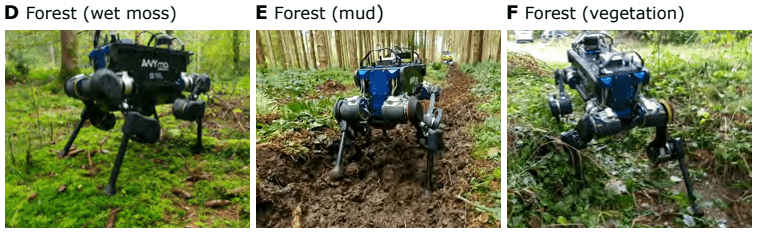We compared the proposed method with the conventional method in three environments, wet moss, mud, and weeds, focusing on the average speed and movement efficiency, respectively. As a result, the proposed method outperforms the conventional method in all combinations of the six points. In addition, it should be noted that the conventional method could not walk in the environment with weeds because its legs were taken up by the weeds, while the proposed method shook off the weeds and walked by itself in such a case.

In addition, although not directly taken into account in the data, the proposed method did not fall over even once while the conventional method fell over many times during the measurement, so we can consider that the proposed method is better than the actual value.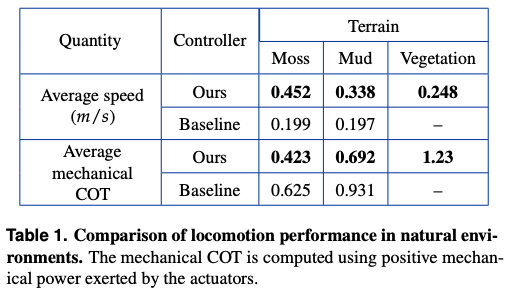DARPA Subterranean Challenge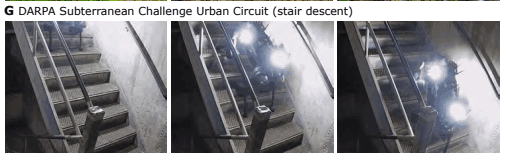The DARPA Subterranean Challenge Urban Circuit is a competition aimed at advancing robotic technology capable of performing tasks in underground environments such as tunnels.

The ANYmal-B with the proposed controller was challenged with four tasks such as descending a steep staircase and successfully continued to operate for 60 minutes without falling over once.

indoor

Finally, I would like to look at an experiment indoors.

In the original paper, comparisons are made under several settings and criteria, but here I would like to present an excerpt concerning the ascent and descent of steps.

The following figure shows the success rate of the proposed method against the width of the step. It is clear from this figure that the proposed method can cope with a wide range of steps even if a weight (10kg) is imposed, while the conventional method has a narrow range of success even without the weight, and it cannot succeed at all with the weight.

This result shows how good the stability of the proposed method is.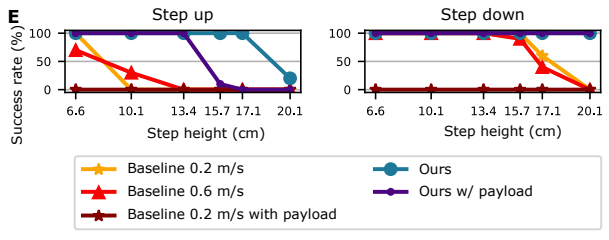technique

Now that we have seen that the proposed method has great performance, let's take a closer look at how the controller was created.

The three points are as follows. I will explain each of them.

• Two-stage strategy learning with a teacher strategy that uses privileged information and a student strategy that uses only intrinsic acceptance information
• Curriculum learning that automatically adjusts the difficulty of the terrain
• Two-stage control structure consisting of generation and tracking of motion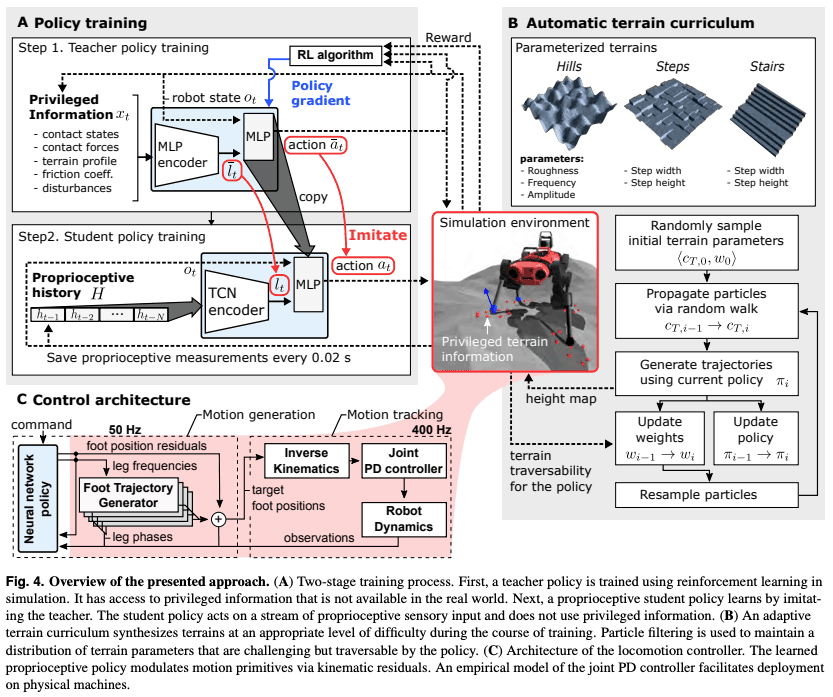Teacher strategies that use privileged information and inherent acceptance Two levels of strategy learning: teacher strategy using privileged information and student strategy using only information

Privileged information refers to information that is useful for walking but difficult to obtain in the real world, such as the force and friction coefficient applied to each leg. In the simulator, the information can be referred to.

Next, proprioceptive information refers to values that can be obtained with high accuracy about the self, such as the position and velocity of each joint.

The main point of this method is to make the robot walk in a real environment where it cannot obtain privileged information, by providing it with rich information to learn the teacher's strategy, and then to learn the student's strategy so that it can walk only with intrinsic receptive information.

Now let's take a closer look at the specific process.

Initially, we train a teacher strategy. We use the usual RL framework, with the assumption that the environment is fully observable once privileged information is incorporated.

Using the privileged information xt and the robot's state ot as input, the robot learns in TRPO to output an appropriate action at. The reward is given according to the speed of approaching the goal.

In this section, we will study not only actions but also latent expressions of privileged information, lt, at the same time. Figure A (upper left) presented at the beginning of this chapter is easy to understand, and you can refer to it as necessary.

We then move on to learning the student measures. The student measures are learned using a sequence of intrinsic receptive information ht, where ht is defined as a subset of the robot's state ot, and H = {ht-1,..., ht-N-1} as the input to the network. where N is a hyperparameter that indicates how far back to consider.

TCN is used as the network structure, and the loss function is defined as follows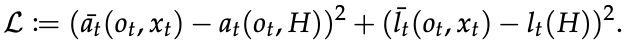where the bar ( ̄ ̄ ̄ ) means that it is the value output from the teacher measure.

It is clear from this equation that student measures learn to imitate the latent representations of action and privileged information given by teacher measures.

We use Temporal Convolutional Network(TCN) for the network structure, which can handle time-series data in the framework of CNN. RNN and GRU are commonly used as the network structure to handle time-series data, but the reason why TCN is used this time is that it has better learning efficiency than GRU, and it can control long data transparently.

Curriculum learning that automatically adjusts the difficulty of the terrain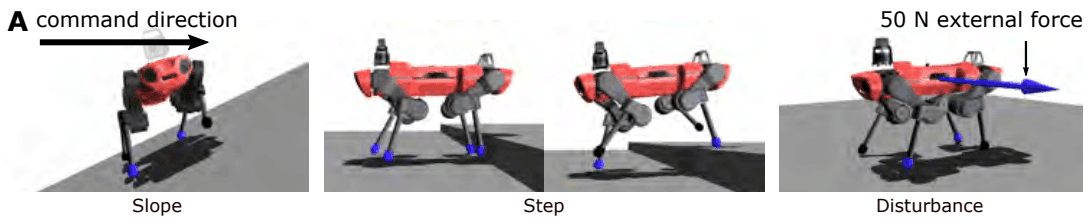The agent mainly learns on three types of terrain (hills, steps, and stairs), but it automatically adjusts the difficulty of the terrain to make learning more efficient. In this section, we will look at the method.

The difficulty of terrain is represented by a vector Ct. Without going into details, the terrain is generated with this Ct as an input. For example, Ct contains information such as the height of steps, and you can see the change of terrain by the difference of parameters in the above figure.

The key point of this method is to approximate the ideal distribution of Ct by using a particle filter.

To think about the ideal Ct, we define a few variables.

Initially, we introduce the following values as a measure of an agent's Traversability under certain measures and terrain.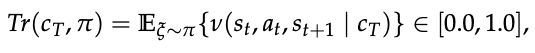Here, v is a variable that represents whether the agent is walking or not, and is set to 1 when the speed is above a certain value, and 0 otherwise. Also, ξ represents the trajectory generated by the strategy.

Next, let us express the goodness of Ct under a measure as follows.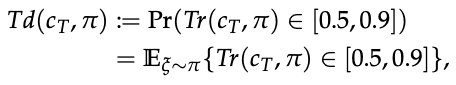As you can see from the equation, we define the goodness of Ct as the probability that the walking ability is between 0.5 and 0.9.

Now that we have a quantitative idea of how good the current terrain parameters are, we will look at an algorithm to sample Ct that will give us a large Td.

To do so, we use a technique called SIR particle filter.

This article does not explain particle filter in detail, but it is a method to approximate probability distribution by particle density. This method is also used for self-position estimation of robots, and there is a lot of information about it on the net, so please refer to it.

Let's look at the flow briefly. First, we prepare Nparticle pairs of Ct and the corresponding weights w. Nparticle is a hyperparameter that represents the number of particles used in the approximation, which is 10 in this paper.

1. Next, the trajectory is generated by Ct and the measures in training, and Td is calculated.
2. The weights are updated to correspond to the obtained Td ratios.
3. Resample Ct and transition it according to the system model.
4. Back to one.

The algorithm is called

This procedure allows for the generation of terrain with an appropriate level of difficulty based on the agent's capabilities.

Two-stage control structure consisting of generation and tracking of motion

The control architecture can be divided into two main parts. One is the motion generator and the other is the motion tracker.

In the motion generation section, the target toe position is output based on the action proposed by the RL agent.

Let's look at the specific computational process. The proposed action at is a 16-dimensional vector, and the part corresponding to one leg has 4 dimensions. It consists of a frequency fi (one dimension) and a correction value Δrfi,T (three dimensions) for each dimension. where i ∈ {1,2,3,4} and corresponds to each leg. Using these values, the target toe position is constructed as follows.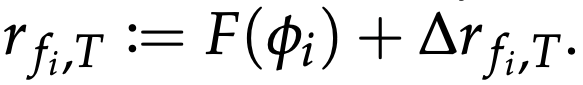Note that F is a function that gives the foot trajectory according to the phase and is a map from [0, 2π) to R3. Φi depends on the frequency fi and the time t and is a variable that represents the phase of each foot.

This gives us our target toe position. Next, we will look briefly at how to achieve it.

In the motion tracking section, the inverse kinematics problem related to the position of the toe position output by the motion generator is solved analytically to calculate the joint angle that gives the toe position. Then, a PD controller is used at each joint to realize the target joint angle.

Future Research Policy

Two future developments of the proposed method are pointed out by the authors.

First, about the fact that the animals could only acquire a gait pattern similar to that of a trot gait (a horse's trot-like gait), the hypothesis that they could acquire a wide variety of gait patterns seen in nature by adding ingenuity to the training method was proposed.

Next, we discuss the use of external perception. The proposed method achieves stable walking without using external sensors such as LiDAR or depth sensors. However, by incorporating these sensors effectively, the proposed method can acquire more practical behaviors such as seeing obstacles and bypassing them.

summary

We have seen the performance of a controller that has achieved stable walking in an unknown environment and the methods that have been used to achieve this.

As I lightly mentioned at the end, it is expected that more and more excellent quadruped robots will be born by using external sensors. I think this is a field in which we can look forward to future development.

If you have any suggestions for improvement of the content of the article,